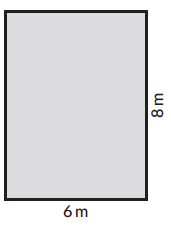## Introduction to Area

Area is the space occupied by a shape/figure. Let’s try and understand this using an example,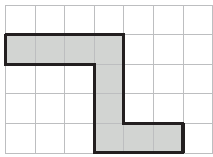The area of this shape is the number of shaded unit squares. Assume that each side of the unit square is 1 cm.

Area = 9 sq.cm

## Formula for calculating area of different shapes

 Shape Formula for perimeter Examples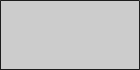$$\text{length} \times \text{breadth}$$\begin{align}A &= 4\,cm \times 2\,cm\\ & = 8\,sq.cm \end{align}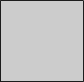$$\text{side} \times \text{side}$$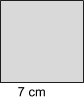\begin{align} A & = 7\,cm \times 7\,cm \\ &= 49\,sq.cm \end{align}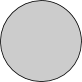\begin{align}\pi r^2 \end{align}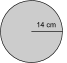\begin{align} A & = {22 \over 7} \times 14\,cm \times 14\,cm \\ & = 616 \,sq.cm \end{align}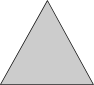\begin{align} {1 \over 2} \times \text{base} \times \text{height} \end{align}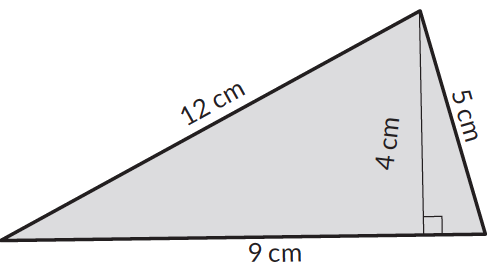\begin{align}A & = {1 \over 2} \times 9\,cm \times 4\,cm \\ &= 18\,sq.cm \end{align}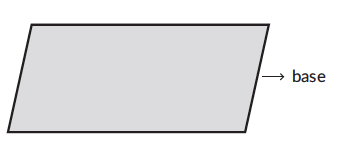$$\text{base} \times \text{height}$$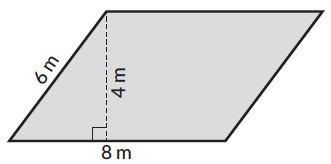\begin{align}A & = 8\,m \times 4\, m \\ & = 32 \, sq.m \end{align}

### Area of a Triangle

An interesting way to understand Area of a Triangle. See how the formula is derived.

### Area of a Trapezium

The parallel sides of the trapezium $$ABCD$$ measure $$a$$ and $$b.$$ The height of the trapezium is $$h.$$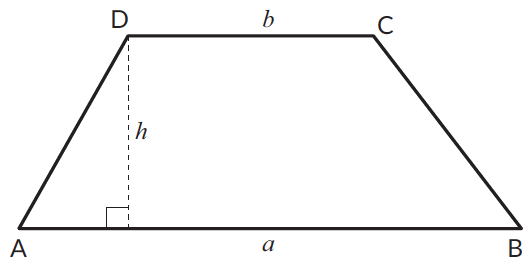We will draw one of the diagonals, say $$BD.$$  $$BD$$ divides the trapezium into two triangles as shown,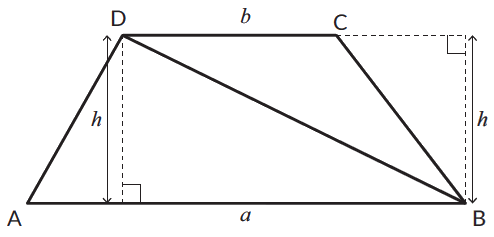\begin{align} \text{Area of trapezium } ABCD & = \text{Area of } \triangle ABC + \text{Area of } \triangle BDC \\& = {1 \over 2} \times b \times h + {1 \over 2} \times b \times h \\ &= {1 \over 2} \times a \times h + {1 \over 2} \times b \times h \\ &= {1 \over 2} \times h\,(a + b) \end{align}

Let’s calculate the area of the given trapezium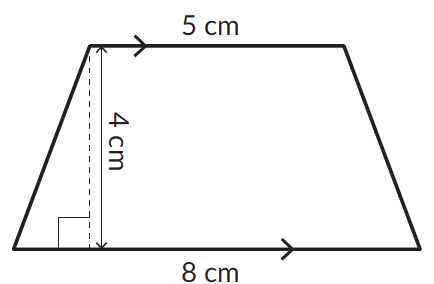\begin{align} \text{Area of trapezium } & = {1 \over 2} \times h (a + b) \\ & = {1 \over 2} \times 4 (5 + 8) \\ & = {1 \over 2} \times 52 \\ & = 26 \, cm^2 \end{align}

### Area of Rhombus

Let’s find the area of a rhombus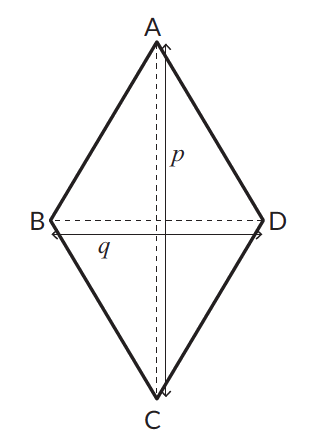The diagonals of the rhombus $$ABCD$$ measure $$p$$ and $$q.$$

The diagonals of a rhombus bisect each other at $$90^\circ .$$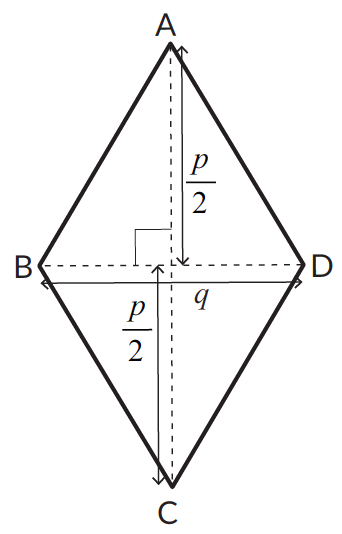\begin{align} \text{The area of the rhombus }ABCD &= \text{Area of } \triangle ABD + \text{Area of } \triangle BCD \\ &= {1 \over 2} \times q \times {p \over 2} + {1 \over 2} \times q \times {p \over 2} \\ &= {1 \over 2 } \times q \times p \end{align}

Let’s calculate the area of the given trapezium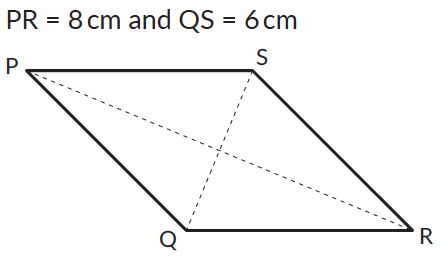\begin{align} \text{Area of trapezium }PQRS & = {1 \over 2} \times PR \times QS \\ & = {1 \over 2} \times 8 \times 6 \\ & = 24\,cm^2 \end{align}

### Area of a kite

Let’s find area of a kite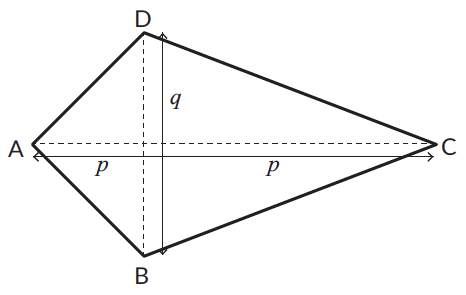$$AC$$ bisects $$BD$$ at $$90^\circ.$$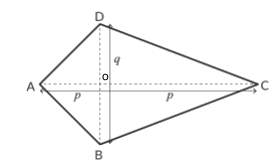Suppose,
$$AO = x$$
$$OC = p - x$$

\begin{align} \text{The area of the rhombus } ABCD & = \text{Area of } \triangle ABD + \text{Area of } \triangle BCD \\ & = {1 \over 2} \times x \times q + {1 \over 2} \times q \times p - x \\ & = {1 \over 2} \times q (x + p - x) \\ & = {1 \over 2} \times q \times p \end{align}

Let’s try and calculate the area of the given kite.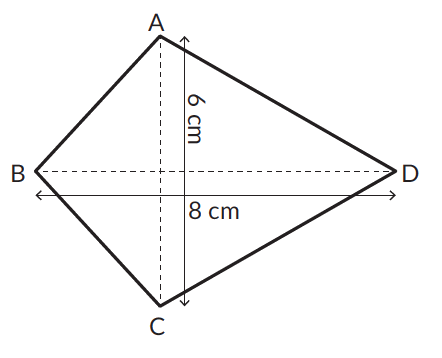## Points to remember while calculating area

• Any one side of a parallelogram can be the base of the parallelogram. The height of a parallelogram is the perpendicular distance between the base and its parallel side.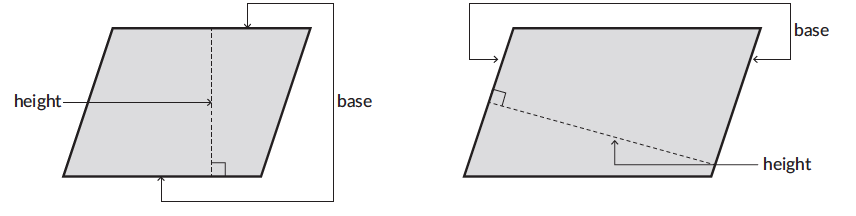• Any side of a triangle can be the base of the triangle. The perpendicular distance between the base and the vertex opposite to the base is the height.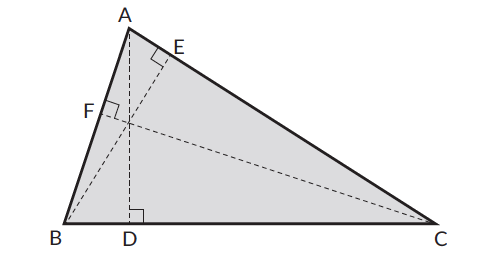## Tips and tricks

• Tip: Children often memorise the formula for calculating area of plane shapes. Help them see how the formula has been derived using the grid lines. For example, in the given shape it is easier to visualise the space or the region occupied by the shape. The rectangle has occupied 9 squares each with an area of 1cm and hence the area of the rectangle is 9sq.cm.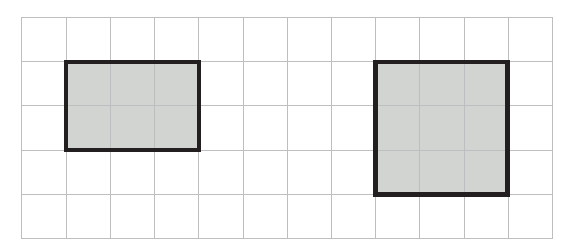• Tip: Children often get confused between area and perimeter as concepts. A thorough understanding can be built by asking the child to trace the surface of any 3D shape and see area is essentially the space or the region covered by a shape.
• For rectilinear shapes doubling the sides doubles the perimeter. For example, the area of the given rectangle is 20 sq.cm. However if the sides double up (length 10 cm and width 8 cm) the area will increase by 4 times, it will be 80 sq.cm.Q1. Calculate the area of,

a.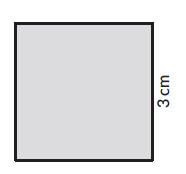b.